# Chapter 5 Normal Probability Distributions LarsonFarber 4 th

• Slides: 25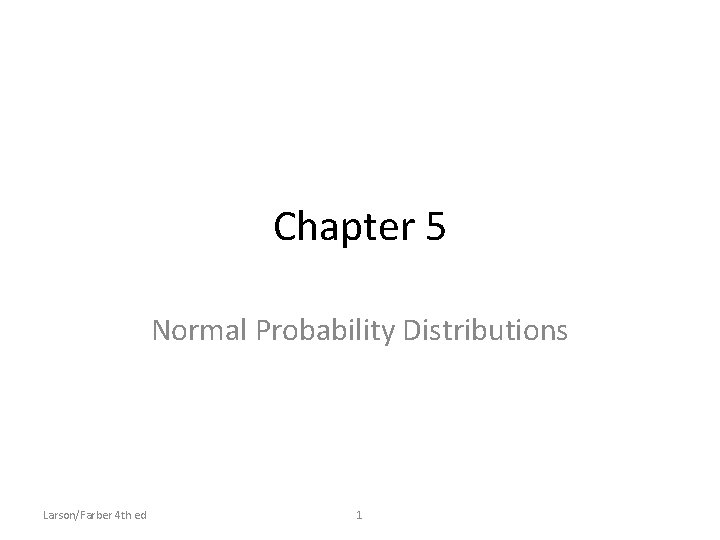Chapter 5 Normal Probability Distributions Larson/Farber 4 th ed 1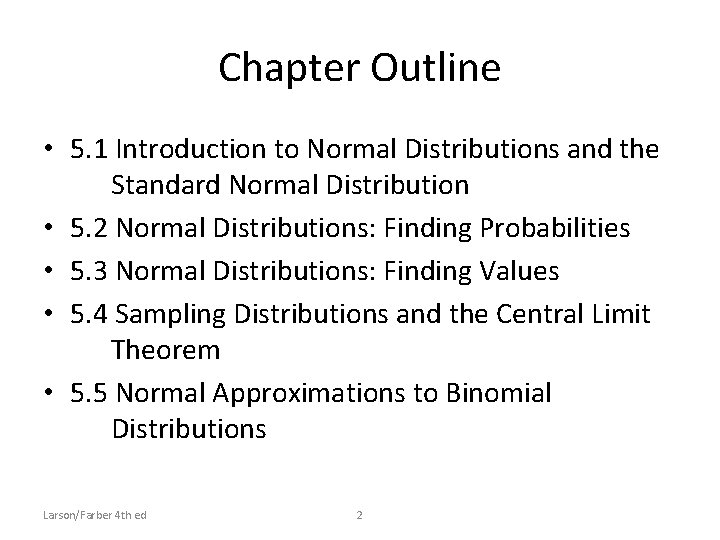Chapter Outline • 5. 1 Introduction to Normal Distributions and the Standard Normal Distribution • 5. 2 Normal Distributions: Finding Probabilities • 5. 3 Normal Distributions: Finding Values • 5. 4 Sampling Distributions and the Central Limit Theorem • 5. 5 Normal Approximations to Binomial Distributions Larson/Farber 4 th ed 2Section 5. 1 Introduction to Normal Distributions Larson/Farber 4 th ed 3Section 5. 1 Objectives • Interpret graphs of normal probability distributions • Find areas under the standard normal curve Larson/Farber 4 th ed 4Properties of a Normal Distribution Continuous random variable • Has an infinite number of possible values that can be represented by an interval on the number line. Hours spent studying in a day 0 3 6 9 12 15 18 21 24 The time spent studying can be any number between 0 and 24. Continuous probability distribution • The probability distribution of a continuous random variable. Larson/Farber 4 th ed 5Properties of Normal Distributions Normal distribution • A continuous probability distribution for a random variable, x. • The most important continuous probability distribution in statistics. • The graph of a normal distribution is called the normal curve. x Larson/Farber 4 th ed 6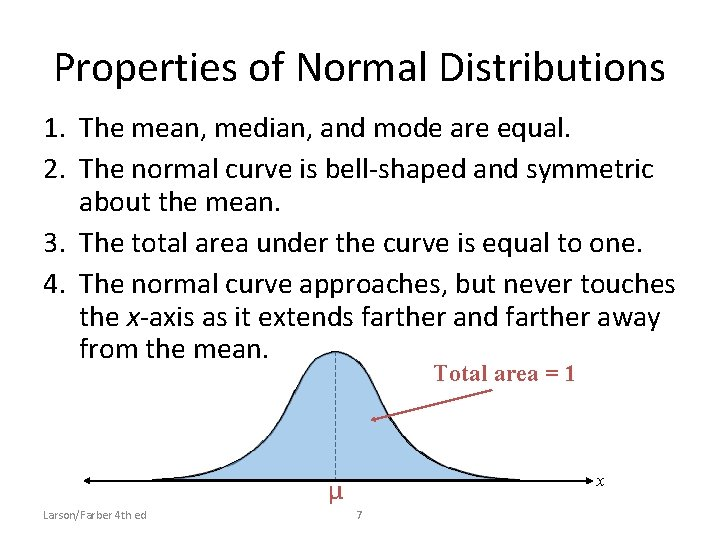Properties of Normal Distributions 1. The mean, median, and mode are equal. 2. The normal curve is bell-shaped and symmetric about the mean. 3. The total area under the curve is equal to one. 4. The normal curve approaches, but never touches the x-axis as it extends farther and farther away from the mean. Total area = 1 x μ Larson/Farber 4 th ed 7Properties of Normal Distributions 5. Between μ – σ and μ + σ (in the center of the curve), the graph curves downward. The graph curves upward to the left of μ – σ and to the right of μ + σ. The points at which the curve changes from curving upward to curving downward are called the inflection points. Inflection points μ 3σ Larson/Farber 4 th ed μ 2σ μ 8 μ+σ μ + 2σ μ + 3σ xMeans and Standard Deviations • A normal distribution can have any mean and any positive standard deviation. • The mean gives the location of the line of symmetry. • The standard deviation describes the spread of the data. μ = 3. 5 σ = 1. 5 Larson/Farber 4 th ed μ = 3. 5 σ = 0. 7 9 μ = 1. 5 σ = 0. 7Example: Understanding Mean and Standard Deviation 1. Which curve has the greater mean? Solution: Curve A has the greater mean (The line of symmetry of curve A occurs at x = 15. The line of symmetry of curve B occurs at x = 12. ) Larson/Farber 4 th ed 10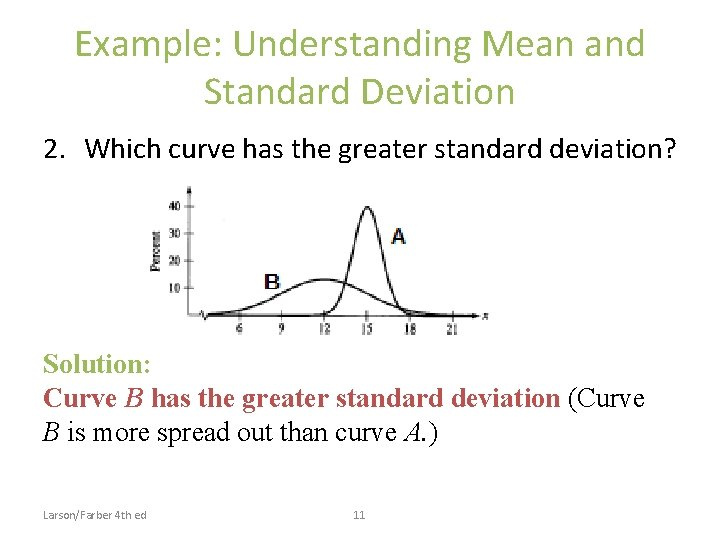Example: Understanding Mean and Standard Deviation 2. Which curve has the greater standard deviation? Solution: Curve B has the greater standard deviation (Curve B is more spread out than curve A. ) Larson/Farber 4 th ed 11Example: Interpreting Graphs The heights of fully grown white oak trees are normally distributed. The curve represents the distribution. What is the mean height of a fully grown white oak tree? Estimate the standard deviation. Solution: σ = 3. 5 (The inflection points are one standard deviation away from the mean) μ = 90 (A normal curve is symmetric about the mean) Larson/Farber 4 th ed 12The Standard Normal Distribution Standard normal distribution • A normal distribution with a mean of 0 and a standard deviation of 1. Area = 1 3 2 1 0 1 2 3 z • Any x-value can be transformed into a z-score by using the formula Larson/Farber 4 th ed 13The Standard Normal Distribution • If each data value of a normally distributed random variable x is transformed into a z-score, the result will be the standard normal distribution. Standard Normal Distribution s s=1 m x m=0 • Use the Standard Normal Table to find the cumulative area under the standard normal curve. Larson/Farber 4 th ed 14 z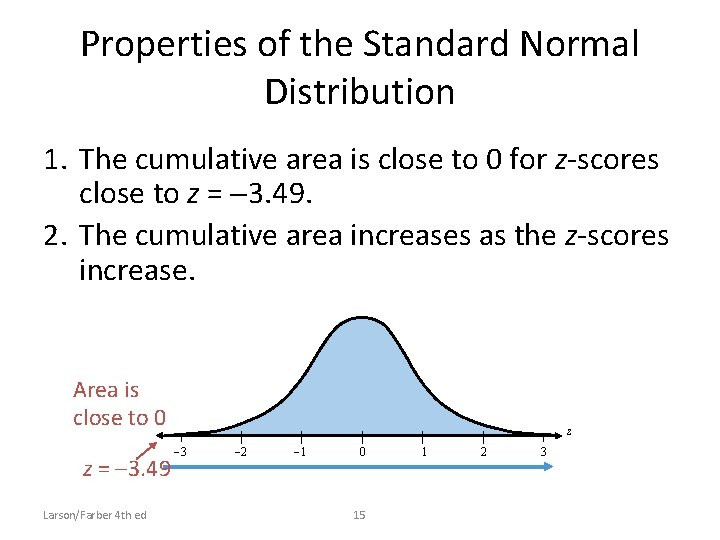Properties of the Standard Normal Distribution 1. The cumulative area is close to 0 for z-scores close to z = 3. 49. 2. The cumulative area increases as the z-scores increase. Area is close to 0 z = 3. 49 Larson/Farber 4 th ed z 3 2 1 0 15 1 2 3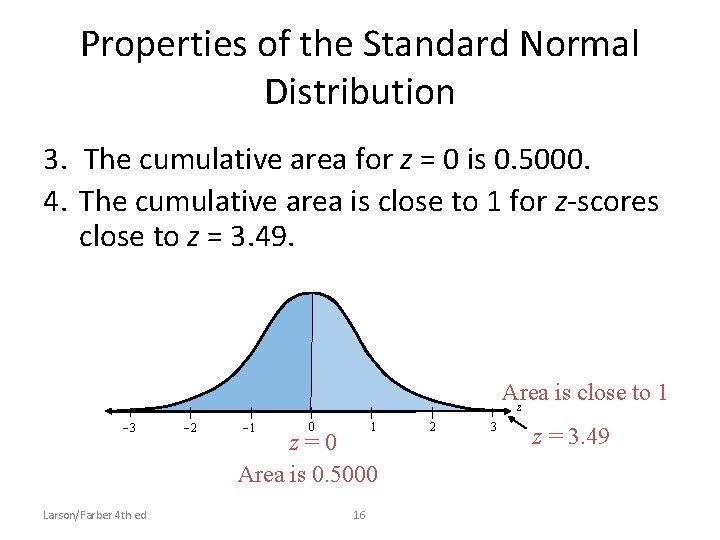Properties of the Standard Normal Distribution 3. The cumulative area for z = 0 is 0. 5000. 4. The cumulative area is close to 1 for z-scores close to z = 3. 49. Area is close to 1 z 3 Larson/Farber 4 th ed 2 1 0 1 z=0 Area is 0. 5000 16 2 3 z = 3. 49Example: Using The Standard Normal Table Find the cumulative area that corresponds to a zscore of 1. 15. Solution: Find 1. 1 in the left hand column. Move across the row to the column under 0. 05 The area to the left of z = 1. 15 is 0. 8749. Larson/Farber 4 th ed 17Example: Using The Standard Normal Table Find the cumulative area that corresponds to a zscore of -0. 24. Solution: Find -0. 2 in the left hand column. Move across the row to the column under 0. 04 The area to the left of z = -0. 24 is 0. 4052. Larson/Farber 4 th ed 18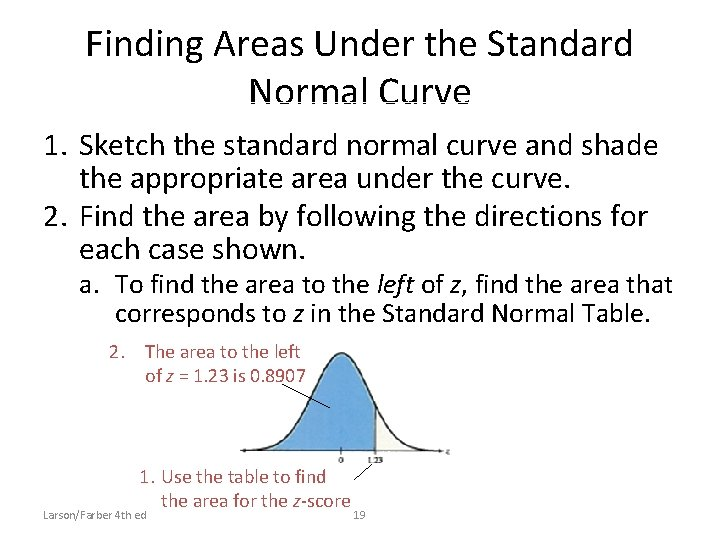Finding Areas Under the Standard Normal Curve 1. Sketch the standard normal curve and shade the appropriate area under the curve. 2. Find the area by following the directions for each case shown. a. To find the area to the left of z, find the area that corresponds to z in the Standard Normal Table. 2. The area to the left of z = 1. 23 is 0. 8907 1. Use the table to find the area for the z-score Larson/Farber 4 th ed 19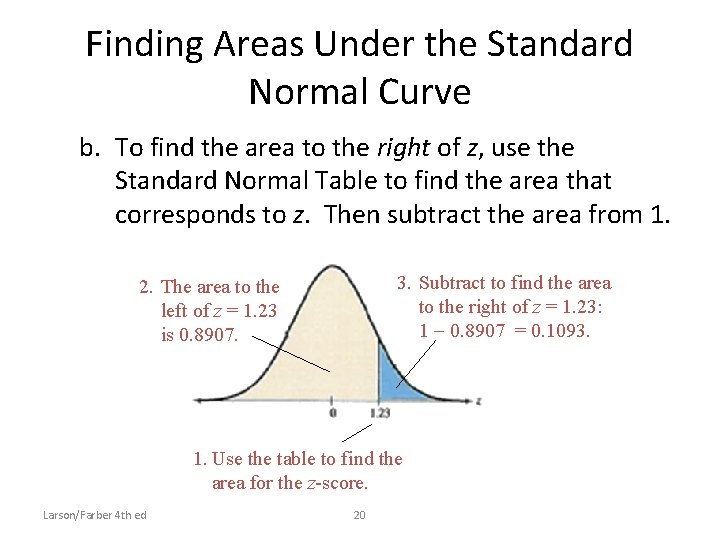Finding Areas Under the Standard Normal Curve b. To find the area to the right of z, use the Standard Normal Table to find the area that corresponds to z. Then subtract the area from 1. 3. Subtract to find the area to the right of z = 1. 23: 1 0. 8907 = 0. 1093. 2. The area to the left of z = 1. 23 is 0. 8907. 1. Use the table to find the area for the z-score. Larson/Farber 4 th ed 20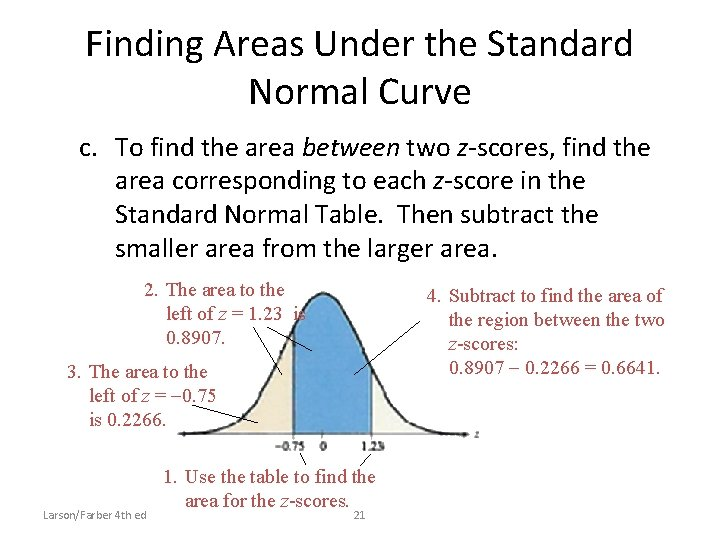Finding Areas Under the Standard Normal Curve c. To find the area between two z-scores, find the area corresponding to each z-score in the Standard Normal Table. Then subtract the smaller area from the larger area. 2. The area to the left of z = 1. 23 is 0. 8907. 4. Subtract to find the area of the region between the two z-scores: 0. 8907 0. 2266 = 0. 6641. 3. The area to the left of z = 0. 75 is 0. 2266. Larson/Farber 4 th ed 1. Use the table to find the area for the z-scores. 21Example: Finding Area Under the Standard Normal Curve Find the area under the standard normal curve to the left of z = -0. 99. Solution: 0. 1611 0. 99 z 0 From the Standard Normal Table, the area is equal to 0. 1611. Larson/Farber 4 th ed 22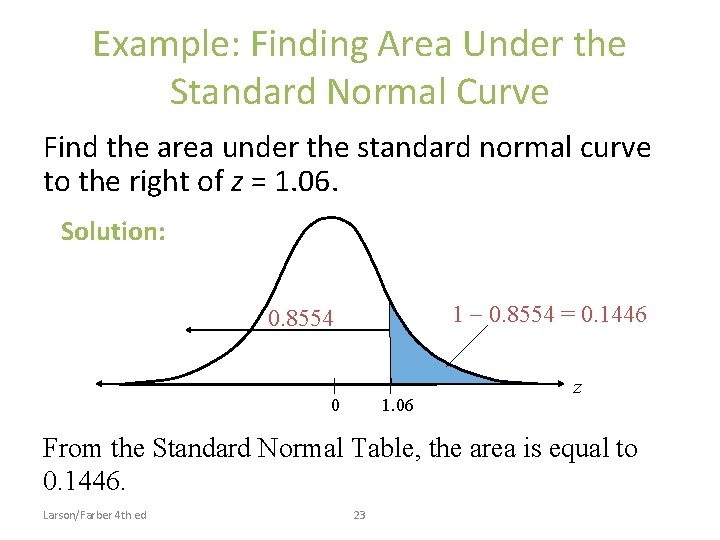Example: Finding Area Under the Standard Normal Curve Find the area under the standard normal curve to the right of z = 1. 06. Solution: 1 0. 8554 = 0. 1446 0. 8554 0 1. 06 z From the Standard Normal Table, the area is equal to 0. 1446. Larson/Farber 4 th ed 23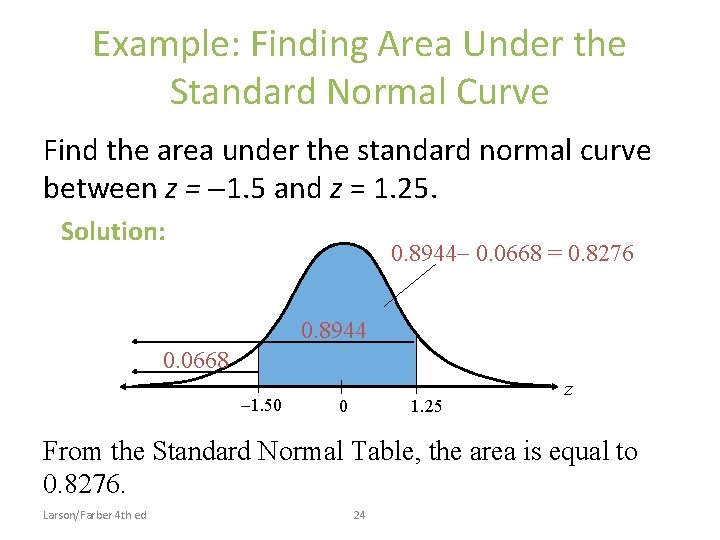Example: Finding Area Under the Standard Normal Curve Find the area under the standard normal curve between z = 1. 5 and z = 1. 25. Solution: 0. 8944 0. 0668 = 0. 8276 0. 8944 0. 0668 1. 50 0 1. 25 z From the Standard Normal Table, the area is equal to 0. 8276. Larson/Farber 4 th ed 24Section 5. 1 Summary • Interpreted graphs of normal probability distributions • Found areas under the standard normal curve Larson/Farber 4 th ed 25